# Trigonometric equations Questions and Answers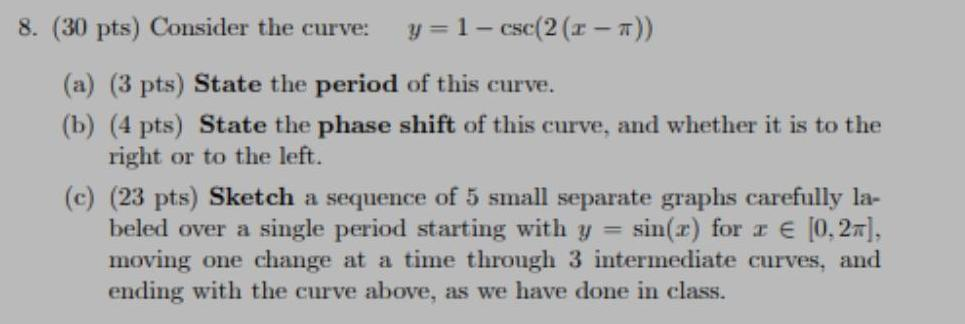Math
Trigonometric equations
Consider the curve: y = 1-csc(2 (x-π)) (a) State the period of this curve. (b) State the phase shift of this curve, and whether it is to the right or to the left. (c) Sketch a sequence of 5 small separate graphs carefully labeled over a single period starting with y = sin(x) for x ∈[0,2π], moving one change at a time through 3 intermediate curves, and ending with the curve above, as we have done in class.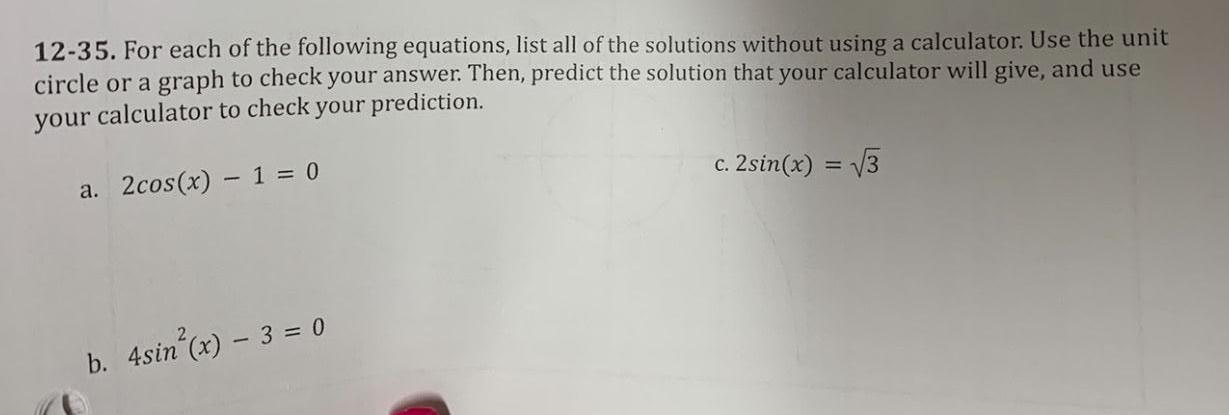Math
Trigonometric equations
12-35. For each of the following equations, list all of the solutions without using a calculator. Use the unit circle or a graph to check your answer. Then, predict the solution that your calculator will give, and use your calculator to check your prediction. a. 2cos(x) - 1 = 0 b. 4sin²(x) - 3 = 0 c. 2sin(x) = √3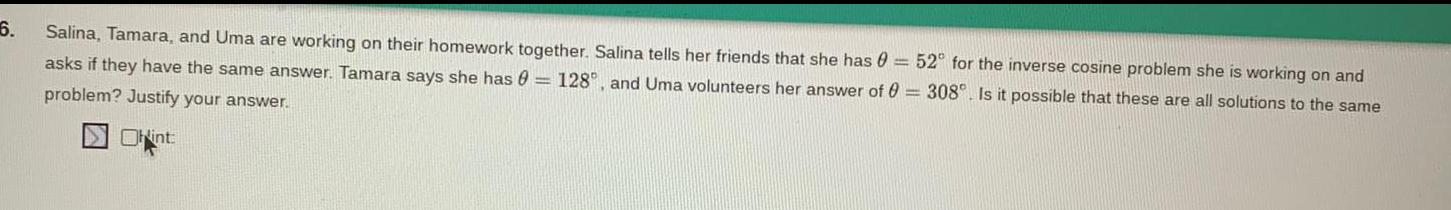Math
Trigonometric equations
Salina, Tamara, and Uma are working on their homework together. Salina tells her friends that she has θ = 52° for the inverse cosine problem she is working on and asks if they have the same answer. Tamara says she has θ = 128°, and Uma volunteers her answer of θ = 308°. Is it possible that these are all solutions to the same problem? Justify your answer.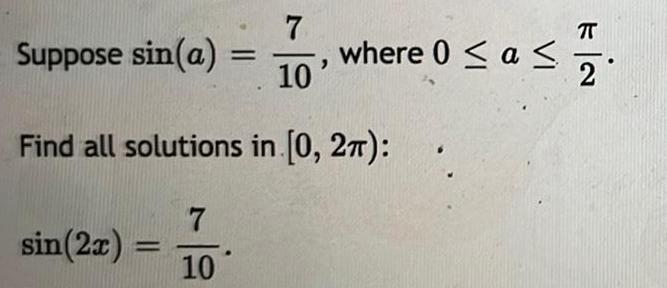Math
Trigonometric equations
Suppose sin(a)= 7/10, where 0 ≤ a ≤ π/2 Find all solutions in [0, 2π): sin(2x) = 7/10Math
Trigonometric equations
Suppose tan(a)=5/8 where 0 ≤ a ≤ π/2 Find all solutions in [0, 2π):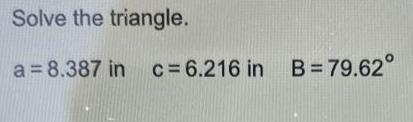Math
Trigonometric equations
Solve the triangle. a = 8.387 in c= 6.216 in B=79.62°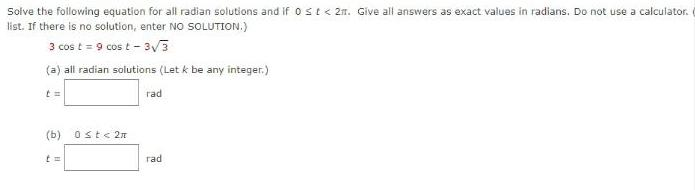Math
Trigonometric equations
Solve the following equation for all radian solutions and if 0 < t <2π. Give all answers as exact values in radians. Do not use a calculator. list. If there is no solution, enter NO SOLUTION.) 3 cos t = 9 cost t-3√3 (a) all radian solutions (Let k be any integer.) t=rad (b) 0≤t <2n t= rad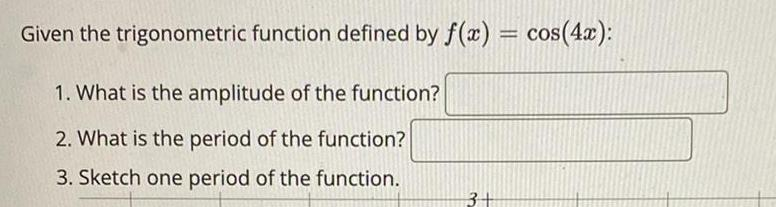Math
Trigonometric equations
Given the trigonometric function defined by f(x) = cos(4x): 1. What is the amplitude of the function? _______ 2. What is the period of the function? ______ 3. Sketch one period of the function.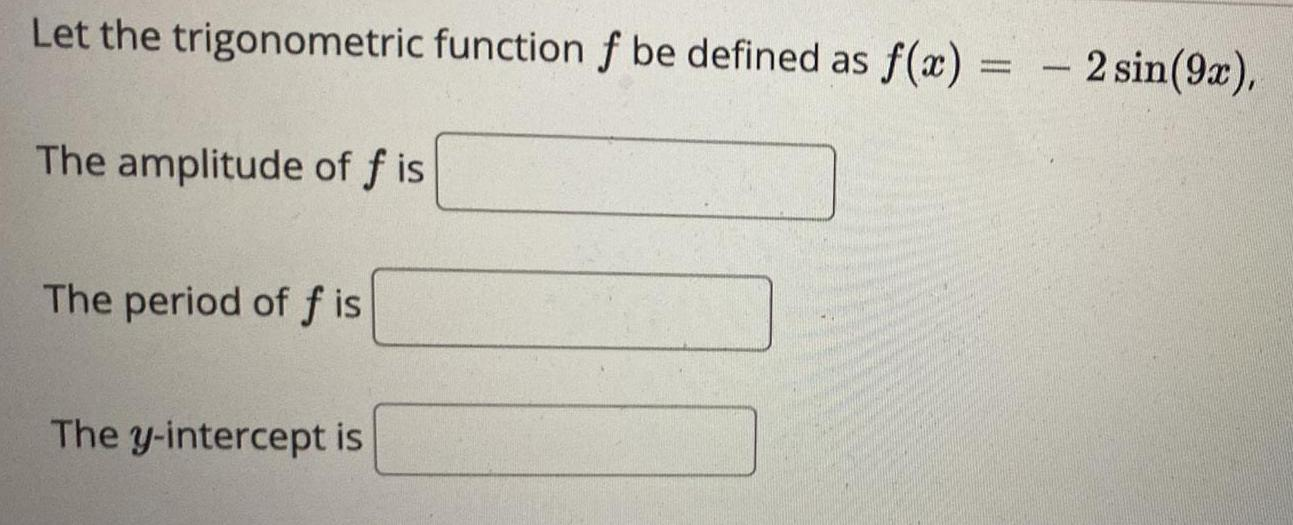Math
Trigonometric equations
Let the trigonometric function f be defined as f(x)= -2sin(9x), The amplitude of f is _____ The period of f is ____ The y-intercept is ____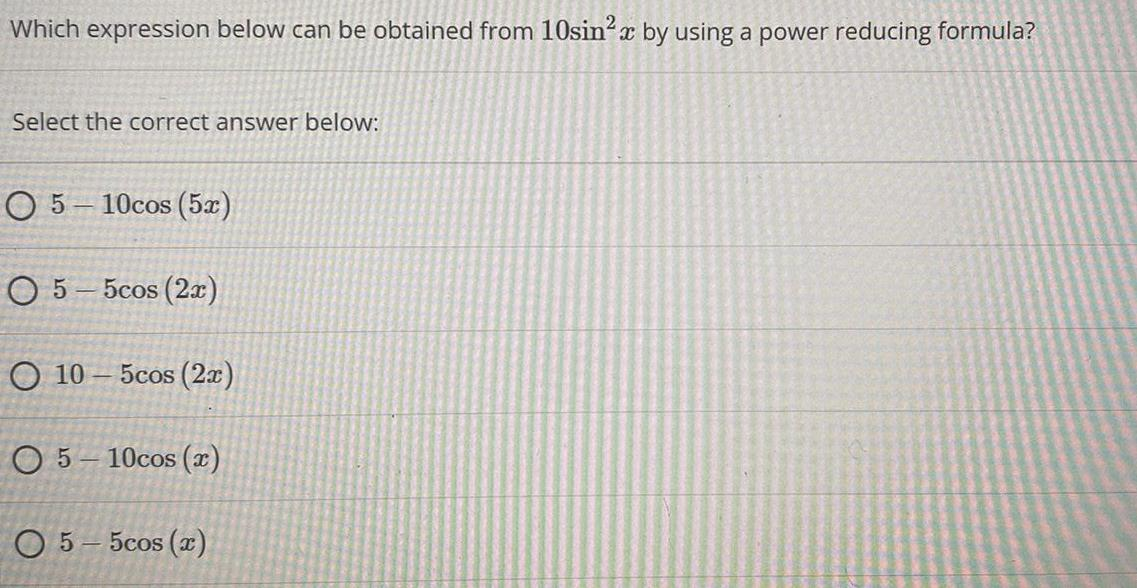Math
Trigonometric equations
Which expression below can be obtained from 10sin²x by using a power reducing formula? Select the correct answer below: 5 - 10cos (5x) 5-5cos (2x) 10-5cos (2x) 5-10cos (x) 5-5cos (x)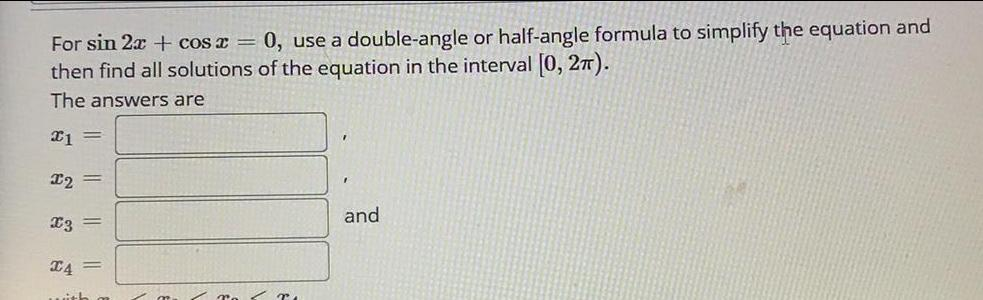Math
Trigonometric equations
For sin 2x + cos x = 0, use a double-angle or half-angle formula to simplify the equation and then find all solutions of the equation in the interval [0, 2π). The answers are x1 = 12 x3 = x4 =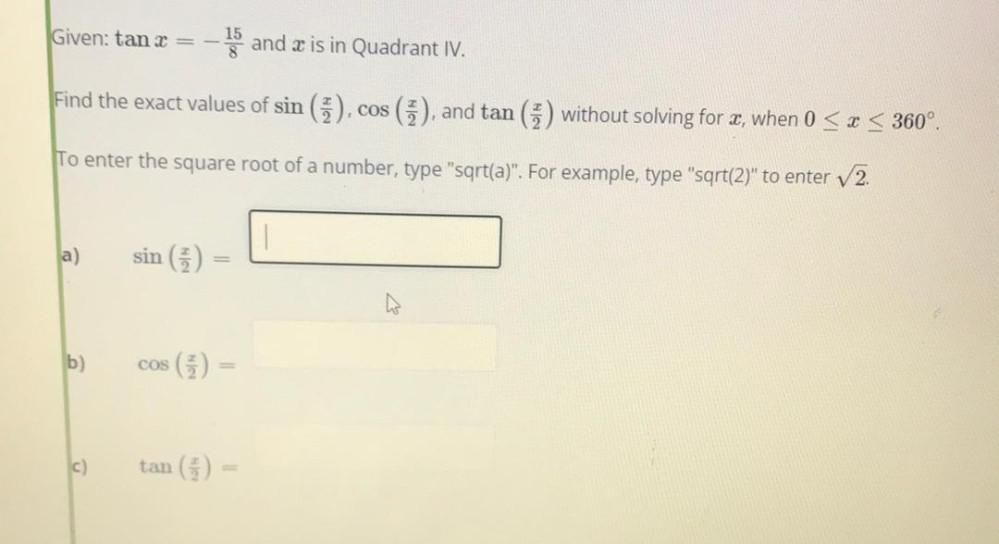Math
Trigonometric equations
Given: tanx = -15/8 and x is in Quadrant IV. Find the exact values of sin (x/2),cos (x/2), and tan (x/2) without solving for x, when 0 ≤ x ≤ 360°. To enter the square root of a number, type "sqrt(a)". For example, type "sqrt(2)" to enter √2. a)sin (x/2)= ___ b)cos (x/2)= ___ c)tan (x/2)= ___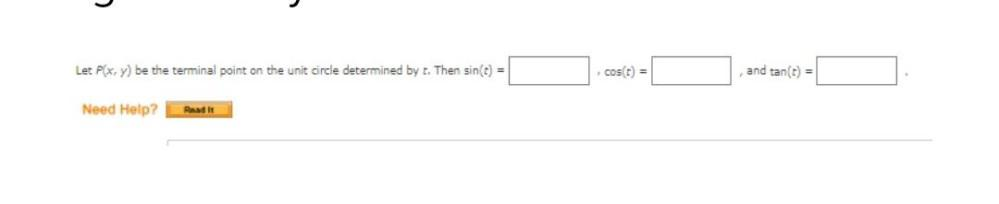Math
Trigonometric equations
Let P(x, y) be the terminal point on the unit circle determined by t. Then sin(t) = ___, cos(t) = ___ , and tan(t) = ___.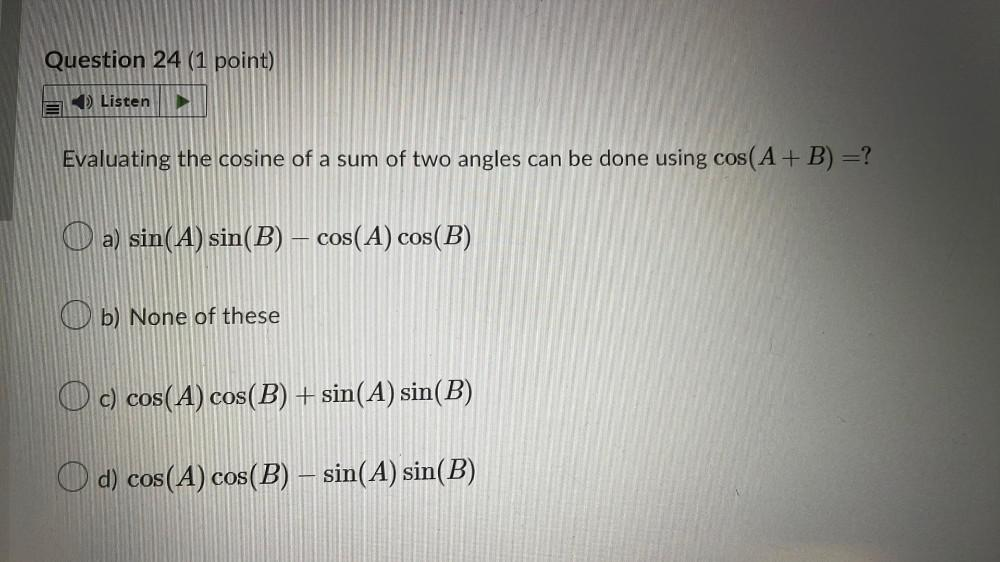Math
Trigonometric equations
Evaluating the cosine of a sum of two angles can be done using cos(A + B) = ? a) sin(A) sin(B) – cos(A) cos(B) b) None of these c) cos(A) cos(B) + sin(A) sin(B) d) cos(A) cos(B) – sin(A) sin(B)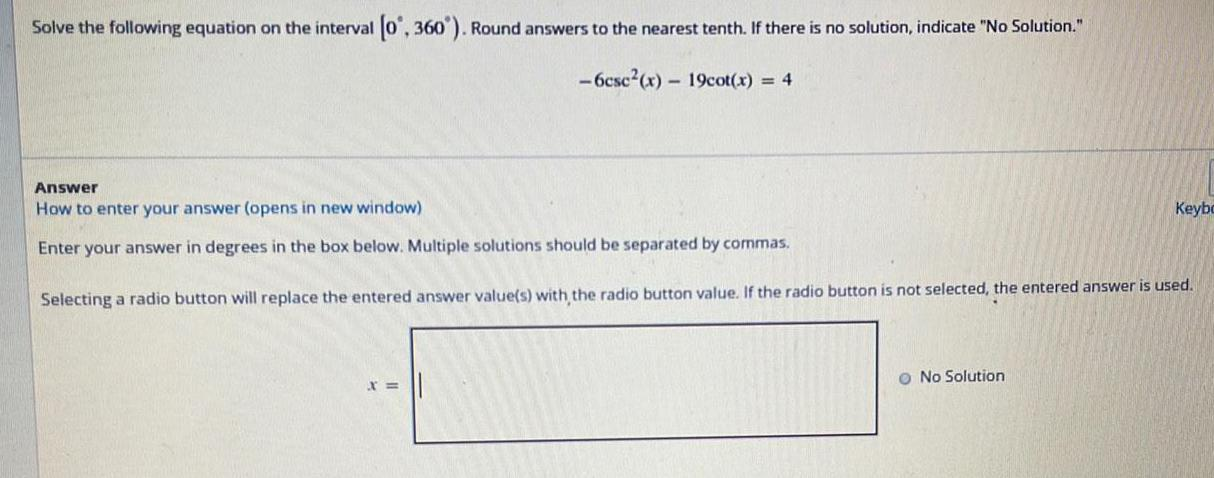Math
Trigonometric equations
Solve the following equation on the interval [0°, 360°). Round answers to the nearest tenth. If there is no solution, indicate "No Solution." -6csc²(x) - 19cot(x) = 4Math
Trigonometric equations
Express the following function as a purely algebraic function. sec(cos-¹(3x))